## Measuring Time: Seconds, 24-hour Clock and Duration

Calculate Time Duration

#### 1. Minutes and Seconds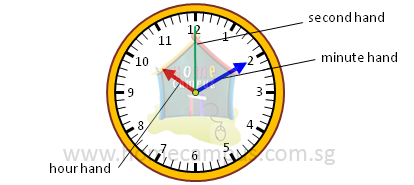The second is a unit of measurement of time. It is smaller than the minute.
We use the second to tell a moment of time more accurately or precisely.
1 minute  =  60 seconds
1 min   =   60 s

We use h to denote hour, min to denote minute and s to denote second.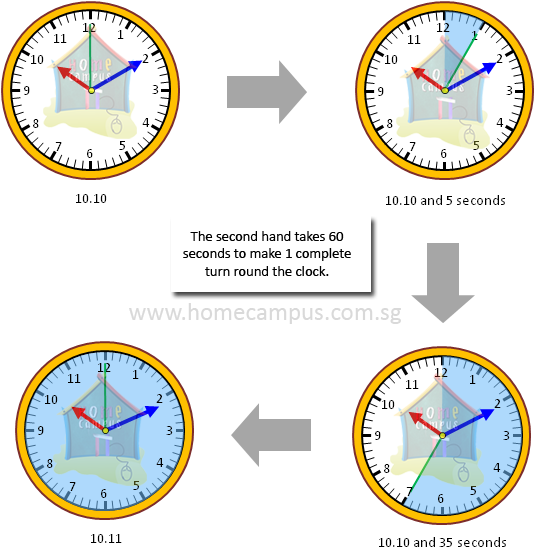#### 2. The 24-Hour Clock

The following time line shows times in 24-hour clock and 12-hour clock.Rachel leaves home for school at 6.45 in the morning.Using the 12-hour clock, we write this time as 6.45 a.m. and read it as six forty-five a.m.

Using the 24-hour clock, we write this time as 06 45 and read it as zero six forty-five hours.
a.m. is the period from midnight to noon.
Rachel returns home from school at 2.25 in the afternoon.Using the 12-hour clock, we write this time as 2.25 p.m. and read it as two twenty-five p.m.

Using the 24-hour clock, we write this time as 14 25 and read it as fourteen twenty-five hours.
p.m. is the period from noon to midnight.

We add 12 hours to the 12-hour clock time in p.m. to change it to 24-hour clock time.
Example: Express 3.45 p.m. using the 24-hour clock.
3  +  12  =  15
3.45 p.m. is 15 45 in the 24-hour clock.

We subtract 12 hours from 24-hour clock time to change it to the 12-hour clock time in p.m.
Example: Express 20 30 using the 12-hour clock.
20  −  12  =  8
20 30 is 8.30 p.m. in the 12-hour clock.

#### 3. In the clock below, where should the missing second hand be at?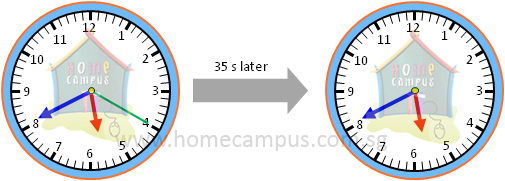35 seconds later, the missing second hand of the clock will be at the position shown below (the green hand is the second hand).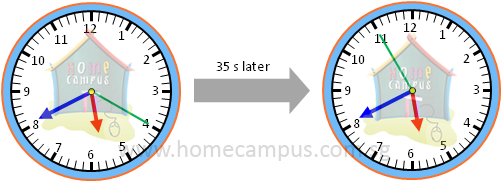#### 4. Find the duration.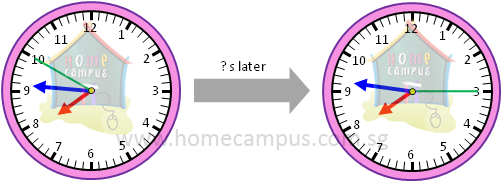The first clock shows a time of 7.45 and 50 s. The second clock shows a time of 7.46 and 15 s.

From 7.45 and 50 s to 7.46, the duration is 10 s.
From 7.46 to 7.46 and 15 s, the duration is 15 s.

10 s + 15 s = 25 s
The duration between the two times is 25 s.

#### 5. The following table shows how Dennis spent last Sunday. Complete the table using the 24-hour clock or the 12-hour clock as appropriate.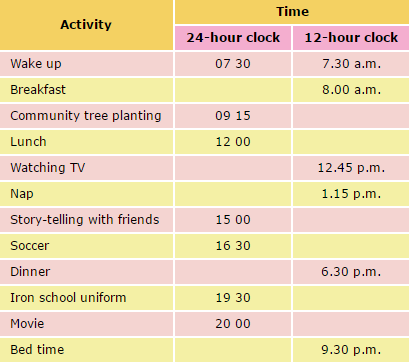Here's the completed table: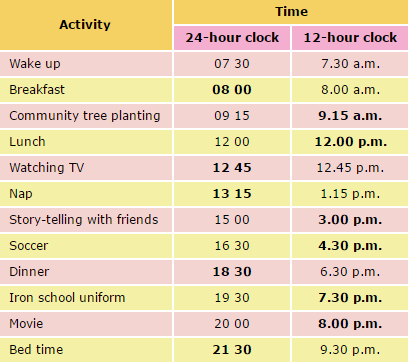#### 6. Derek started typing a composition at 09 30 and finished typing at 10 45. How long did he take to type the composition? a) Express your answer in minutes. b) Express your answer in hours and minutes.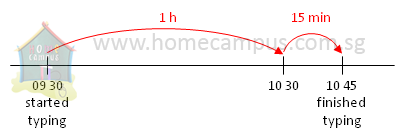a) From 09 30 to 10 45, the duration is 1 h 15 min.
Derek took 1 h 15 min to type the composition.

b) From 09 30 to 10 45, the duration is 75 minutes.
Derek took 75 minutes to type the composition.

#### 7. Mikey entered the library at 16 45 and left 1 h 30 min later. At what time did he leave the library? a) Express your answer in 12-hour clock. b) Express your answer in 24-hour clock.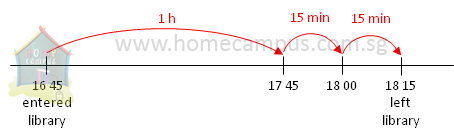30 min = 15 min + 15 min
a) 1 h 30 min after 16 45 is 18 15.
Using 24-hour clock, he left the library at 18 15.

b) 18 15 is 6.15 p.m. in 12-hour clock.
Using 12-hour clock, he left the library at 6.15 p.m.

#### 8. The train from Sunshine City to Moonlight City took 2 1 2 h. If it left Sunshine City at 10.30 a.m., what time did it reach Moonlight City? a) Express your answer in 12-hour clock. b) Express your answer in 24-hour clock.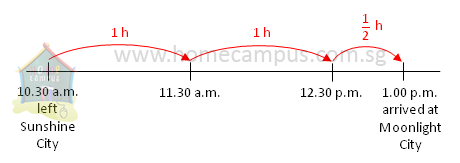2 h after 10.30 a.m. is 12.30 p.m.
 1 2
h after 12.30 p.m. is 1.00 p.m.

a)
 2 1 2
h after 10.30 a.m. is 1.00 p.m.
Using 12-hour clock, the train reached Moonlight City at 1.00 p.m.

b) 1.00 p.m. is 13 00 in 24-hour clock.
Using 24-hour clock, the train reached Moonlight City at 13 00.

#### 9. Rayan took an overnight flight from Mumbai to Singapore. The flight took 5 h 40 min to arrive in Singapore at 04 15. At what time did the flight leave Mumbai?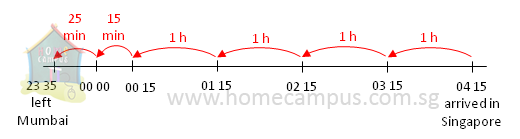Count backwards.
40 min = 15 min + 25 min
5 h 40 min before 04 15 is 22 35.
The flight left Mumbai at 22 35.